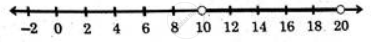# Solve the inequation:–1<3 –x5≤1 - Mathematics and Statistics

Sum

Solve the inequation:
– 1 < 3  – "x"/5 ≤ 1

#### Solution

– 1 < 3  – "x"/5 ≤ 1

Multiplying the inequation by 5, the sign of inequality changes
5 > – 15 + x > – 5
Adding 15 on both the sides, we get
20 > x > 10
⇒ 10 < x < 20
The solution contains all the real values of x lying between 10 and 20, excluding the boundary value.
The solution set can be written in the form of open interval (10, 20).Concept: Solution of Linear Inequality
Is there an error in this question or solution?
Chapter 8: Linear Inequations - Exercise 8.1 [Page 116]

#### APPEARS IN

Balbharati Mathematics and Statistics 2 (Commerce) 11th Standard HSC Maharashtra State Board
Chapter 8 Linear Inequations
Exercise 8.1 | Q 4. (vi) | Page 116
Share